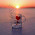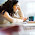# Data Structure and Algorithm Analysis Set 30

## Data Structure and Algorithm Analysis

### Questions 291 to 300

291.
The mathematical definition for Omega can be defined as, provided f,g:NàR+ and c is a positive constant and n > n0,
(a)   f(n) ≥ c. g(n)  n
(b)   f(n) £ c. g(n)  n
(c)   f(n) ≥ c + g(n)  n
(d)   f(n) £ c + g(n)  n
(e)   f(n) £   g(n)  n.
292.
The q notation is __________
I.     Symmetric.
II.     Reflexive.
III.    Transitive.
(a)  Only (I) above
(b)  Only (II) above
(c)   Only (III) above
(d)   Both (I) and (II) above
(e)   All (I), (II) and (III) above.
293.
From the following pick the one which does not belongs to the same paradigm to which others belongs to.
(a)   Minimum & Maximum problem
(b)   Knapsack problem
(c)   Selection problem
(d)   Merge sort
(e)   Quick sort.
294.
The OBST algorithm in worst case takes _______ time if all c(i, j )’s and r(i, j)’s are calculated.
(a)  O(log n)            (b)  O(n4)                 (c)  O(n3)                  (d)  O(n log n)          (e)  O(n2).
295.
Read the following statements carefully, and choose the correct answer
I.     For the Backtracking algorithms stack data structure is used.
II.     For the Branch-and-bound algorithms queue data structure is used.
(a)   (I) is FALSE but (II) is TRUE
(b)   (I) and (II) both are FALSE
(c)   (I) is TRUE but (II) is FALSE
(d)   (I) and (II) both are TRUE
(e)   (II) is TRUE and (I) can’t be defined.
296.
The weighted array used in TVS problems for the following binary tree is  __________

(a)   [1,2,3,0,0,4,0,5,6]
(b)   [1,2,3,0,0,4,0,5,0,0,0,0,6]
(c)   [1,2,3,4,5,6]
(d)   [1,2,3,0,0,4,5,6]
(e)   [1,3,5,2,4,6].
297.
Let f, t: N→ R+, then t (n) Î Ω (f (n)), iff  f(n) Î O (t (n)) is known as __________
(a)  Limit rule                                         (b)  Rule of inference                             (c)  Duality rule
(d)  Rule of consequences                     (e)  Rule of symmetricity.
298.
Let f, t: N→R ≥ 0, and t (n) ÎO (f (n)) iff  t(n) ≤  c.f (n) where c is positive real constant and n ≥ no, then no is ___________
(a)  Upper bound    (b)  Lower bound                                   (c)  Duality value
(d)  Threshold value (e)  Maximum value.
299.
For the 15-puzzle problem if the initial arrangement is as follows, then the value of ‘x’ used to find the reachability is ________
 1 3 5 7 9 11 14 2 4 8 6 10 12 15 13
(a)  0                      (b)  1                       (c)  8                       (d)  14                     (e)  13.
300.
The time taken by nondeterministic sorting algorithm is ______
(a)  O(1)                  (b)  O(log n)            (c)  O(n2)                 (d)  O(n log n)          (e)  O(n).

#### Answers

291.
Answer :  (a)
Reason:  According to the definition of the Omega notation.
292.
Answer :  (e)
Reason:  According to the asymptotic notational rules, q notation is equivalence one
293.
Answer :  (b)
Reason:  The remaining all belongs to divide and conquer paradigm.
294.
Answer :  (c)
Reason:  Because of the memory functions in OBST we calculate c(i, j )’s, r(i, j)’s and w(i , j)’s.
295.
Answer :  (d)
Reason:  According to the need of the traverse, Backtracking algorithm uses stack and Branch-and-bound algorithms uses queue.
296.
Answer :  (b)
Reason:  For any null node in the full binary tree it stores zeros.
297.
Answer :  (c)
Reason:  According to the asymptotic notational rules.
298.
Answer :  (d)
Reason:  According to the definition of Big Oh ( O )
299.
Answer :  (a)
Reason:
 1 3 5 7 9 11 14 2 4 8 6 10 12 15 13

Accordingly the EMPTY cell is there in non shaded position and hence value of ‘x’ is 0, otherwise value of ‘x’ is 1.
300.
Answer :  (e)
Reason:  As there it sorts the entries in one loop. Because in nondeterministic sorting algorithms the abstractions are used which can’t be programmed.

<< Prev

#### 6 comments :

1.nice....i think it will help for NET/SET or any other objective exam....

2.thanks .....it help me to prepared my final exam

3.4.Thanks, nice compilation

5.Algorithms MCQs Questions and Answers
http://gate-exam.in/CS/Syllabus/Computer-Science-Information-Technology/Algorithms

6.